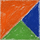xjpvictor's Blog

# wordpress之gravatar缓存

``<img alt='{\$safe_alt}' src='{\$out}' class='avatar avatar-{\$size} photo' height='{\$size}' width='{\$size}' />``

``````function my_get_avatar(\$text) {
preg_match('/https:\/\/secure.gravatar.com\/avatar\/([a-z0-9]+)\?s=(\d+)(?:\S*)&r=(\w*)/',\$text,\$match);
\$ourl = \$match;
\$email_hash = \$match;
\$size = \$match;
\$rate = \$match;
\$ch = curl_init("http://en.gravatar.com/{\$email_hash}.php");
curl_setopt(\$ch,CURLOPT_RETURNTRANSFER,true);
curl_setopt(\$ch,CURLOPT_CONNECTTIMEOUT,10);
curl_exec(\$ch);
\$info = curl_getinfo(\$ch,CURLINFO_HTTP_CODE);
curl_close(\$ch);
if ( \$info === 404 ) {
\$url = '/wp-content/themes/gravatar/default_'.\$size;
} else {
if ( empty(\$rate) ) {
\$rate = "G";
}
\$file = \$_SERVER["DOCUMENT_ROOT"].'/wp-content/themes/gravatar/'.\$email_hash.'_'.\$size.'_'.\$rate;
if ( !file_exists(\$file) ) {
\$img = file_get_contents("https://secure.gravatar.com/avatar/{\$email_hash}?s={\$size}&d=mm");
file_put_contents(\$file,\$img);
}
\$url = '/wp-content/themes/gravatar/'.\$email_hash.'_'.\$size.'_'.\$rate;
}
return preg_replace('/src=\'\S+\'/',"src='{\$url}'",\$text);
}

Update 2013-01-31:

### 一条评论

1.谢谢你 试试看这个代码效率如何

回复